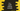# C++ setfill method example## C++ setfill method example:

setfill method is defined in header. This method is used to set a fill character for a stream. In this post, we will learn how to use setfill with an example.

### Definition of setfill:

setfill is defined as like below:

``setfill(char_type c)``

It takes only one parameter, c, which is the fill character for the stream. char_type is the type of characters used by the stream.

This method doesn’t return anything.

## Example of setfill:

Let’s take a look at the below example program:

``````#include <iostream>
#include <iomanip>
using namespace std;

int main()
{
cout << setfill('\$') << setw(5);
cout << "10" << endl;
}``````

In this program, we are using setfill to use \$ as the fill character. setw is used to give the width as 5. So, if anything is printed using cout of length less than 5, it will add \$ to its left.

Since 10 is of size two, it will add three \$ to its left.

The above program will print:

``\$\$\$10``